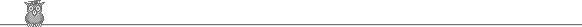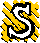### 40th Putnam 1979A1.  Find the set of positive integers with sum 1979 and maximum possible product.A2.  R is the reals. For what real k can we find a continuous function f : R → R such that f(f(x)) = k x9 for all x.A3.  an are defined by a1 = α, a2 = β, an+2 = anan+1/(2an - an+1). α, β are chosen so that an+1 ≠ 2an. For what α, β are infinitely many an integral?A4.  A and B are disjoint sets of n points in the plane. No three points of A ∪ B are collinear. Can we always label the points of A as A1, A2, ... , An, and the points of B as B1, B2, ... , Bn so that no two of the n segments AiBi intersect?A5.  Show that we can find two distinct real roots α, β of x3 - 10x2 + 29x - 25 such that we can find infinitely many positive integers n which can be written as n = [rα] = [sβ] for some integers r, s.A6.  Given n reals αi ∈ [0, 1] show that we can find β ∈ [0, 1] such that ∑ 1/|β - αi| ≤ 8n ∑1n 1/(2i - 1).B1.  Can we find a line normal to the curves y = cosh x and y = sinh x?B2.  Given 0 < α < β, find limλ→0 ( ∫01 (βx + α(1 - x) )λ dx )1/λ.B3.  F is a finite field with n elements. n is odd. x2 + bx + c is an irreducible polynomial over F. For how many elements d ∈ F is x2 + bx + c + d irreducible?B4.  Find a non-trivial solution of the differential equation F(y) ≡ (3x2 + x - 1)y'' - (9x2 + 9x - 2)y' + (18x + 3)y = 0. The solution of F(y) = 6(6x + 1) such that f(0) = 1, and ( f(-1) - 2)( f(1) - 6) = 1 is y=f(x). Find a relation of the form ( f(-2) - a)( f(2) - b) = c.B5.  A convex set S in the plane contains (0, 0) but no other lattice points. The intersections of S with each of the four quadrants have the same area. Show that the area of S is at most 4.B6.  zi are complex numbers. Show that |Re[ (z12 + z22 + ... + zn2)1/2 ]| ≤ |Re z1| + |Re z2| + ... + |Re zn|.To avoid possible copyright problems, I have changed the wording, but not the substance, of all the problems. The original text and official solutions were published in American Mathematical Monthly 87 (1980) 635-40. They are also available (with the solutions expanded) in: Gerald L Alexanderson et al, The William Lowell Putnam Mathematical Competition, 1965-1984. Out of print, but in some university libraries.

Putnam home